This document contains R versions of the boxed examples from Chapter 8 of the “Analysis and Interpretation of Freshwater Fisheries Data” book. Some sections build on descriptions from previous sections, so each section may not stand completely on its own. More thorough discussions of the following items are available in linked vignettes:

The following additional packages are required to complete all of the examples (with the required functions noted as a comment and also noted in the specific examples below).

> library(FSA)          # Subset, capHistSum, mrOpen, removal
> library(Rcapture)     # desc, closedp, profileCI, openp

In addition, external tab-delimited text files are used to hold the data required for each example. These data are loaded into R in each example with read.table(). Before using read.table() the working directory of R must be set to where these files are located on your computer. The working directory for all data files on my computer is set with

> setwd("C:/aaaWork/Web/fishR/BookVignettes/aiffd2007")

In addition, I prefer to not show significance stars for hypothesis test output, reduce the margins on plots, alter the axis label positions, and reduce the axis tick length. In addition, contrasts are set in such a manner as to force R output to match SAS output for linear model summaries. All of these options are set with

> options(width=90,continue=" ",show.signif.stars=FALSE,
contrasts=c("contr.sum","contr.poly"))
> par(mar=c(3.5,3.5,1,1),mgp=c(2.1,0.4,0),tcl=-0.2)

## 8.1 Estimation of Abundance and Density Based on Distance Sampling

An investigator snorkels along a 100-m transect that is randomly located in a stream reach containing 500 m$$^{2}$$. Thirty Brook Trout (Salvelinus fontinalis) are observed at the right-angle distances (m) from the center of the transect. The data is entered below and shown in the text Box. The investigator would like to estimate the density of Brook Trout in the section and the total population in the reach.

The following variables are defined:

Variable Definition
$$n$$ number of animals observed
$$N$$ total population in reach
$$A$$ total area of reach (m$$^2$$)
$$D$$ density of fish (number/m$$^2$$)
$$L$$ length of transect (m)
$$y$$ right angle distance (m) from transect for each animal
$$w$$ effective strip width
$$V(N)$$ estimated variance of population estimate
$$CI$$ confidence interval

### 8.1.1 Preparing Data

The perpendicular distances were entered directly into a vector by including the values in c(). The total number of observations is found by giving the vector of measurements to length(). Finally, the constants values for the transect length and the total area sampled, as provided by the authors, are also entered into objects.

> y <- c(0.7,0.1,0.6,0.3,0.4,0.1,3.2,0.4,0.6,1.4,0.2,0.1,2.5,0.4,4.6,2.2,0.5,1.6,
0.4,0.4,1.5,0.8,0.0,0.2,2.1,0.4,0.4,0.1,1.1,0.6)
> ( n <- length(y) )
 30
> L <- 100
> A <- 500

### 8.1.2 Calculations - Exponential Decline in Sightability

The computational “formulas” for the exponential decline in sightability are shown below. Note that sum() sums the values in the provided vector, sqrt() finds the square root of the provided value, and qnorm() finds the normal deviate that corresponds to the provided lower-tail areas.

> ( w <- sum(y)/(n-1) )
 0.962069
> ( D <- n/(2*L*w) )
 0.155914
> ( N <- D*A )
 77.95699
> ( V <- n/((n/N)^2)*(1-(n/N)+(n/(n-2))) )
 341.6656
> ( CI <- N + qnorm(c(0.025,0.975))*sqrt(V) )
  41.72863 114.18535

### 8.1.3 Calculations - Half-Normal Decline in Sightability

The computational “formulas” for the half-normal decline in sightability are shown below.

> ( w <- 1/sqrt(2/(pi*sum((y^2)/n))) )
 1.752699
> ( D <- n/(2*L*w) )
 0.08558229
> ( N <- D*A )
 42.79115
> ( V <- n/((n/N)^2)*(1-(n/N)+(n/(n-2))) )
 83.64071
> ( CI <- N + qnorm(c(0.025,0.975))*sqrt(V) )
 24.86624 60.71605

### 8.1.4 A Simplifying Function

One of the beauties of R is that repetitive calculations can be “coded” into a function to simplify those calculations. Functions are created in R with function(). The arguments to function() are the arguments that will be required by the function being created. The results of function() are assigned to an object that will be the name of the function being created. The calculations are then contained within a “{” and a “}” and the last line is the object that is returned by the function. For example, the following function, called dstnc(), performs the computations from above when given the perpendicular distances, length of the transect, total area sampled, the distribution type for the decline in sightability, and the level of confidence.

> dstnc <- function(y,L,A,type=c("Exponential","Half-Normal"),conf.level=0.95) {
type <- match.arg(type)
if (type=="Exponential") { w <- sum(y)/(n-1) }
else { w <- 1/sqrt(2/(pi*sum((y^2)/n))) }
D <- n/(2*L*w)
N <- D*A
V <- n/((n/N)^2)*(1-(n/N)+(n/(n-2)))
CI <- N + qnorm(c((1-conf.level)/2,1-(1-conf.level)/2))*sqrt(V)
list(type=type,w=w,D=D,N=N,V=V,CI=CI)
}

For example,

> dstnc(y,L,A)                      # Exponential decline in sightability
$type  "Exponential"$w
 0.962069

$D  0.155914$N
 77.95699

$V  341.6656$CI
  41.72863 114.18535
> d1 <- dstnc(y,L,A,"Half-Normal")  # Half-normal decline in sightability
> round(d1$N,0)  43 > round(d1$CI,0)
 25 61

## 8.2 Applications of Likelihood Functions in Population Estimation

Here, we illustrate the ideas underlying likelihood functions in the context of estimating population size. For this example, consider the situation in which 60 fish are present in a pool within a stream and we have a 40% chance of catching each fish with one electrofishing pass. In this example, we theoretically could catch between 0 and 60 fish. Assuming that the probability a fish is caught is independent among fish, the probability that a specific number of fish will be caught in one pass is given by a binomial probability distribution.

### 8.2.1 Binomial Distribution Calculation Example

In the first paragraph of the box, the authors illustrate using the binomial distribution to compute the probability of observing a catch of 20 fish if the population contains a total of 60 fish and the probability of capture (i.e., the catchability) is 0.4. In R, dbinom() returns this probability when given the observed sample size of “successes” (i.e., fish was caught) as the first argument, the total population size in the size= argument and the probability of “success” in the prob= argument. For example, the illustrative example in the box is computed below.

> N <- 60
> p <- 0.4
> dbinom(20,size=N,prob=p)
 0.06161054

The authors also created a figure (Figure 8.3A) that showed the probability of capturing all possible catches between 0 and 60, given N=60 and q=0.4. This graphic can be constructed with the commented code below.

> catch <- seq(0,60)                         # create all values between 0 and 60
> pr <- dbinom(catch,size=N,prob=p)          # find probabilities for all possible catches
> ( max.catch <- catch[which(pr==max(pr))] ) # find catch with maximum probability
 24
> plot(pr~catch,type="l",xlab="Number Caught",ylab="Probablity")
> abline(v=max.catch,lty=2,col="red")        # mark the maximum catch
> axis(1,max.catch,max.catch,col="red")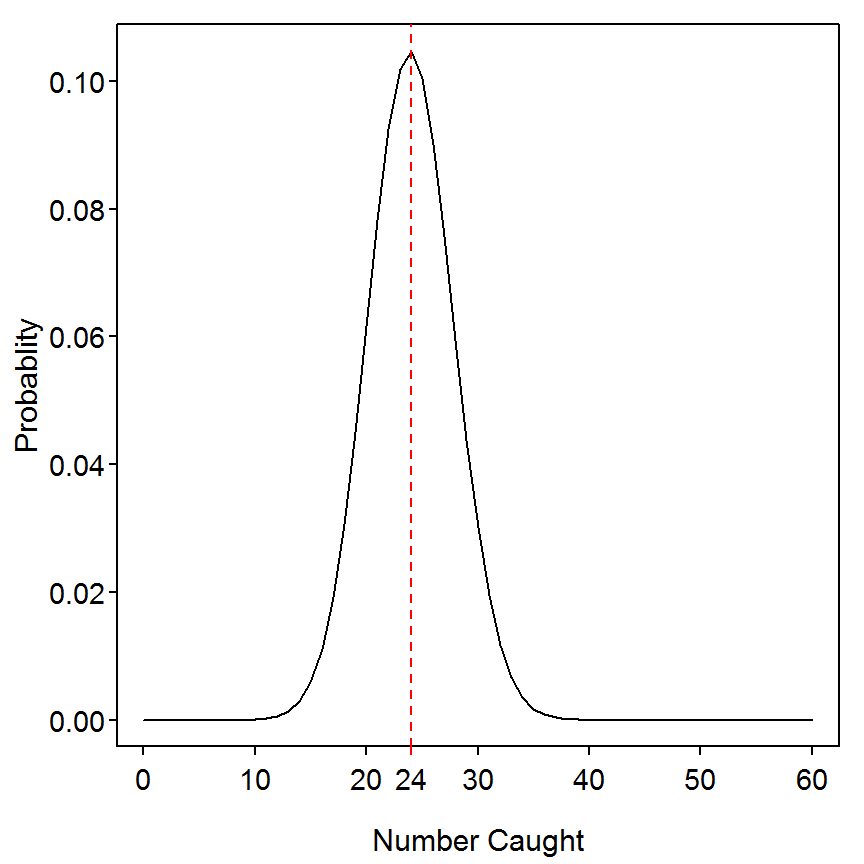### 8.2.2 Binomial Distribution Likelihood Calculation Example

The simple calculation shown in the third paragraph of the box is given as an example of a likelihood calculation is the same as the simple calculation shown above. The calculation (and graphic) of the likelihood of observing n=20 fish given a catchability of 0.4 and a variety of reasonable values of N can be made with the code below.

> possN <- seq(0,100)                        # create possible values of N between 0 and 100
> lh <- dbinom(20,size=possN,prob=p)         # find probabilities for possible Ns
> ( max.lh <- possN[which(lh==max(lh))] )    # find possN with maximum lh
 49 50
> plot(lh~possN,type="l",xlab="Population Size",ylab="Likelihood")
> abline(v=max.lh,lty=2,col="red")           # mark the maximum possN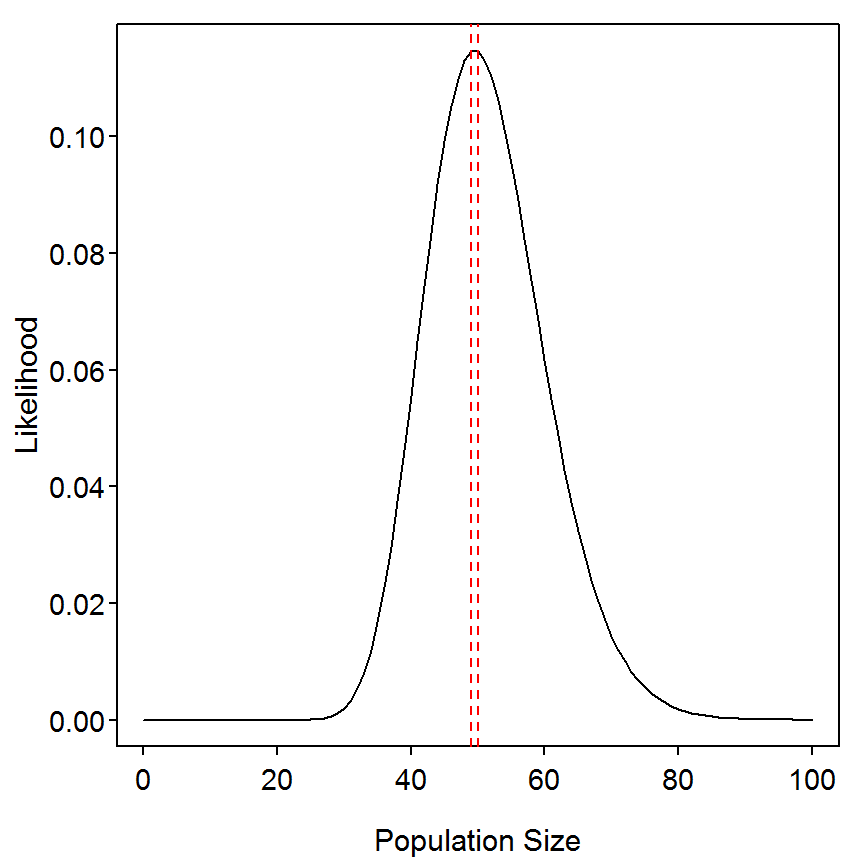The remainder of the discussion and computations in the box are basically repeated in Box 8.5.

## 8.3 Estimation of Population Abundance for a Closed Population Based on the Mark-Recapture Models of Otis et al. (1978)

An investigator conducts a mark-recapture study on a closed population of Largemouth Bass (Micropterus salmoides) in a farm pond in order to determine the abundance of adult fish. The sampling consists of four sampling events; fish captured in each event are given a uniquely numbered Floy tag and released. The capture-recapture data are arranged into a capture matrix in which each cell of the matrix ($$X_{ij}$$) is referenced by fish $$i$$ in row $$i$$ and sample period $$j$$ in column $$j$$. An entry of $$1$$ in the matrix indicates that a fish was caught, and a $$0$$ indicates that the fish was not caught during that sampling period. Fish 1, for example (see box in text), was caught in all four sampling periods, whereas fish 4 was caught in only the first sample period.

### 8.3.1 Preparing Data

The Box8_3.txt data file is read and the structure is observed.

> d3 <- read.table("data/Box8_3.txt",header=TRUE)
> str(d3)
'data.frame':   20 obs. of  5 variables:
$Fish : int 1 2 3 4 5 6 7 8 9 10 ...$ time1: int  1 1 1 1 1 1 1 0 0 0 ...
$time2: int 1 1 0 0 1 0 0 1 1 1 ...$ time3: int  1 0 1 0 0 1 0 1 0 0 ...
$time4: int 1 0 0 0 1 1 0 0 0 1 ... The fish identification numbers in the first column are not needed for any further analysis. They can be dropped with the remaining columns saved to another object with, > ( d3ch <- d3[,-1] )  time1 time2 time3 time4 1 1 1 1 1 2 1 1 0 0 3 1 0 1 0 4 1 0 0 0 5 1 1 0 1 6 1 0 1 1 7 1 0 0 0 8 0 1 1 0 9 0 1 0 0 10 0 1 0 1 11 0 1 0 0 12 0 1 0 0 13 0 1 1 1 14 0 0 1 0 15 0 0 1 0 16 0 0 1 0 17 0 0 1 1 18 0 0 0 1 19 0 0 1 1 20 0 0 0 1 ### 8.3.2 Summarized Capture Histories The descriptive() function from the Rcapture package. A description of the use of Rcapture can be obtained by typing vignette("RcaptureJSS","Rcapture"). can be used to compute some basic summary values from the capture histories. This function requires the matrix or data frame containing just the capture histories as the first argument. Two graphics useful for identifying different types of capture probability heterogeneities (see Table 1 on page 4 of the Rcapture vignette) by submitting the saved descriptive object to plot(). > ( desc <- descriptive(d3ch) )  Number of captured units: 20 Frequency statistics: fi ui vi ni i = 1 10 7 2 7 i = 2 6 6 4 9 i = 3 3 5 5 10 i = 4 1 2 9 9 fi: number of units captured i times ui: number of units captured for the first time on occasion i vi: number of units captured for the last time on occasion i ni: number of units captured on occasion i > plot(desc)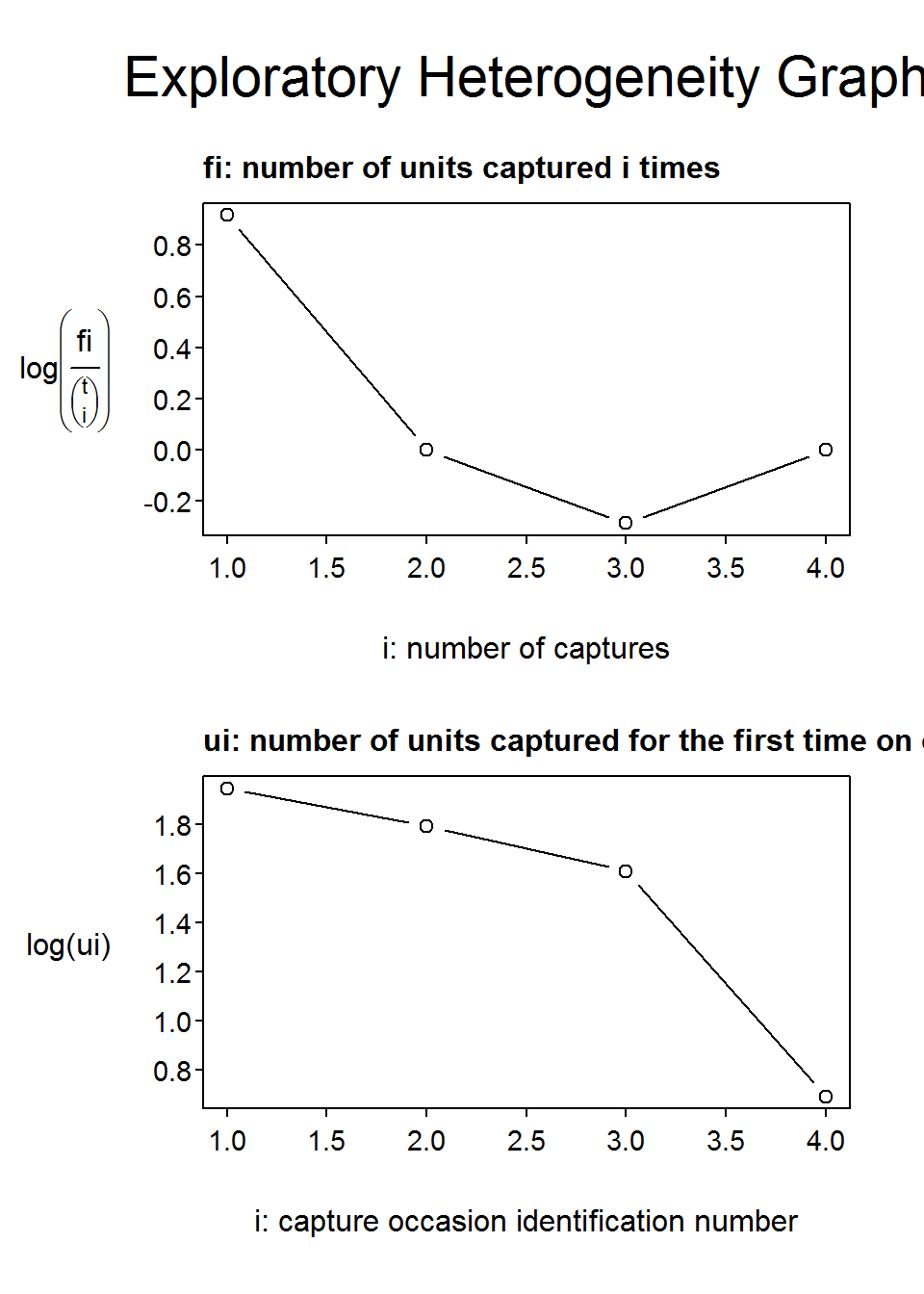The capHistSum() function from, the FSA package, can also be used to provide basic summary values from the capture history. As with descriptive(), capHistSum() requires the matrix or data frame containing just the capture histories as the first argument (consult the help – ?capHistSum – for the meanings of the output). > capHistSum(d3ch) $caphist

0001 0010 0011 0100 0101 0110 0111 1000 1010 1011 1100 1101 1111
2    3    2    3    1    1    1    2    1    1    1    1    1

$sum n m R M u v f 1 7 0 7 0 7 2 10 2 9 3 9 7 9 4 6 3 10 5 10 13 5 10 3 4 9 7 0 18 2 9 1$methodB.top
i=1 i=2 i=3 i=4
j=1  NA   3   2   0
j=2  NA  NA   3   2
j=3  NA  NA  NA   5
j=4  NA  NA  NA  NA

$methodB.bot i=1 i=2 i=3 i=4 m 0 3 5 7 u 7 6 5 2 n 7 9 10 9 R 7 9 10 0$m.array
ni c2 c3 c4 not recapt
i=1  7  3  2  0          2
i=2  9 NA  3  2          4
i=3 10 NA NA  5          5
i=4  9 NA NA NA          9

attr(,"class")
 "CapHist"

### 8.3.3 Model Fitting

The suite of models (with a few additions) of Otis et al. (1978) can be efficiently fit with closedp() from the Rcapture package. This function requires the matrix or data frame containing just the capture histories as the first argument. The results from the model fits can be seen by appending $results to the saved closedp object. As in Box 8.3, the AIC values suggest that the simplest model, M0, appears to be the best fit. With this model, the estimated population size is 24. > ( res1 <- closedp(d3ch) )  Number of captured units: 20 Abundance estimations and model fits: abundance stderr deviance df AIC BIC infoFit M0 23.8 2.9 5.995 13 39.819 41.811 OK Mt 23.7 2.8 5.122 10 44.947 49.925 OK Mh Chao (LB) 26.2 5.3 4.901 11 42.726 46.709 OK Mh Poisson2 27.2 6.4 4.944 12 40.769 43.756 OK Mh Darroch 31.4 13.6 4.902 12 40.727 43.714 warning #1 Mh Gamma3.5 37.7 27.5 4.905 12 40.730 43.717 warning #1 Mth Chao (LB) 26.2 5.3 3.977 8 47.802 54.772 OK Mth Poisson2 27.1 6.3 4.021 9 45.846 51.820 OK Mth Darroch 31.4 13.6 3.977 9 45.802 51.777 warning #1 Mth Gamma3.5 37.9 27.9 3.981 9 45.806 51.780 warning #1 Mb 27.3 8.9 5.508 12 41.332 44.320 OK Mbh 24.1 9.1 5.236 11 43.060 47.043 OK > res1$results
              abundance    stderr deviance df      AIC      BIC infoFit
M0             23.81181  2.854140 5.994536 13 39.81934 41.81081       0
Mt             23.72945  2.815307 5.121960 10 44.94677 49.92543       0
Mh Chao (LB)   26.25000  5.327797 4.901391 11 42.72620 46.70913       0
Mh Poisson2    27.18408  6.356713 4.943792 12 40.76860 43.75580       0
Mh Darroch     31.41107 13.589402 4.901897 12 40.72670 43.71390       1
Mh Gamma3.5    37.66371 27.454294 4.905021 12 40.72983 43.71702       1
Mth Chao (LB)  26.16814  5.268197 3.976915  8 47.80172 54.77185       0
Mth Poisson2   27.12167  6.308089 4.020844  9 45.84565 51.82004       0
Mth Darroch    31.44878 13.634755 3.977369  9 45.80218 51.77657       1
Mth Gamma3.5   37.92454 27.870193 3.980964  9 45.80577 51.78016       1
Mb             27.29994  8.917304 5.507532 12 41.33234 44.31954       0
Mbh            24.05249  9.113156 5.235598 11 43.06041 47.04333       0

The AIC values are somewhat different from what is presented in the box. However, if one rescales the AIC values for the three models presented in the box so that the AIC for the M0 model is the same as that presented in the box then one can see that the differences between what is presented here and in the box is largely a constant value (with some rounding error).

> ( res1a <- res1$results[c("M0","Mt","Mb"),"AIC"] )  M0 Mt Mb 39.81934 44.94677 41.33234  > res1a-min(res1a)+26.598  M0 Mt Mb 26.59800 31.72542 28.11100  The 95% confidence interval for the abundance based on the profile likelihood (with a corresponding graphic) is found by submitting the capture history matrix or data frame as the first argument and the abbreviation of the “best” model in the m= argument to profileCI() from the Rcapture package. > ( CI1 <- profileCI(d3ch,m="M0") )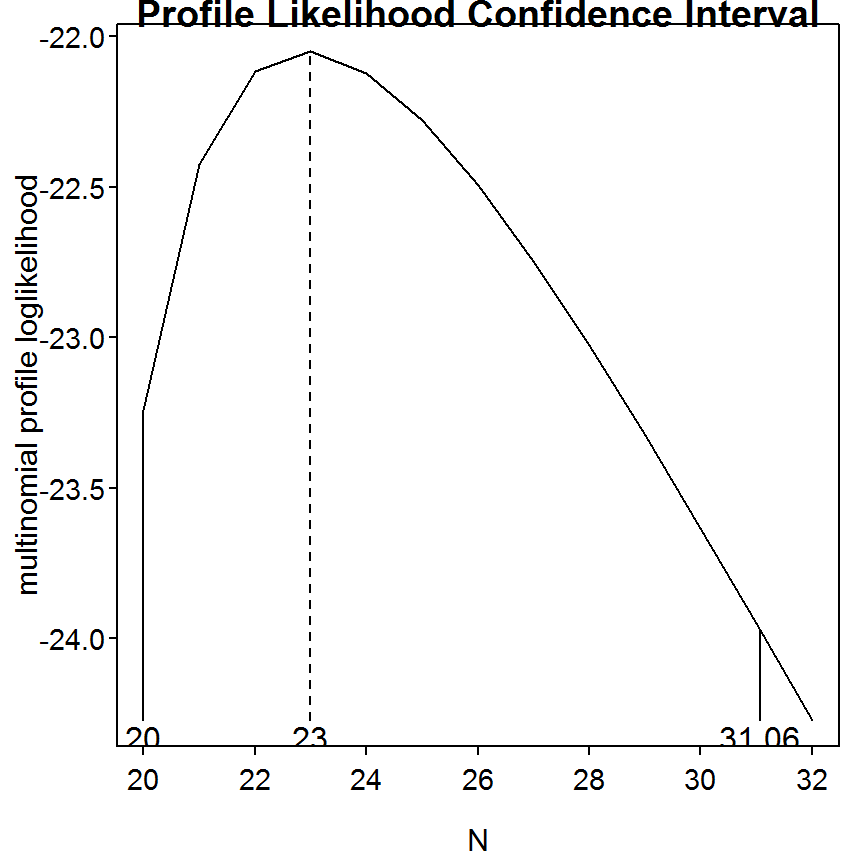Number of captured units: 20 95% profile likelihood confidence interval: abundance InfCL SupCL M0 23 20 31.06255 ## 8.4 Estimation of Abundance Based on a Cormack-Jolly-Seber Method for Open Populations In order to determine the conservation status of Desert Pupfish, Cyprinodon macularius, a graduate student performs a 3-year capture-recapture experiment on the population in a desert pool that is closed to immigration and emigration but where recruitment and mortality occur on an annual basis. ### 8.4.1 Preparing Data The Box8_4.txt data file is read and the structure is observed. Note that the fish identification numbers in the first column are not needed for any further analysis and that the rest of the columns contain the capture histories. > d4 <- read.table("data/Box8_4.txt",header=TRUE) > str(d4) 'data.frame': 30 obs. of 4 variables:$ ID   : int  1 2 3 4 5 6 7 8 9 10 ...
$time : int 1 1 1 1 1 1 1 1 1 1 ...$ time2: int  1 1 1 1 0 0 0 0 0 0 ...
$time3: int 1 1 0 0 1 1 1 1 0 0 ... ### 8.4.2 Analysis Using the FSA Package The analyses in this vignette do not exactly follow those shown in box because special purpose packages are available in R for these analyses (unlike SAS). One of these packages is FSA. A more thorough description of the technique used in FSA can be found in the Chapter 7 of the Introductory Fisheries Analysis with R book. It should be noted that the results here will not exactly match those in the Box because the code in the FSA uses formulas that have been modified to guard against bias in the parameter estimates, which were not used in the Box. The capHistSum() function is used to provide basic summary values from the capture histories. This function requires the matrix or data frame containing the capture histories as the first argument. If the data frame has columns that do not contain capture history information, as the first column in this example, then the specific columns containing the capture history must be identified in the cols2use= argument. In this example, we simply want to exclude the first column, so cols2use=-1 can be used. Of particular interest in the computation of the Jolly-Seber method is the summaries in the so-called “Method B table.” These summaries are found in mb.top and mb.bot of the object saved from capHistSum(). > chsum <- capHistSum(d4,cols2use=-1) > chsum$methodB.top
    i=1 i=2 i=3
j=1  NA   4   4
j=2  NA  NA   6
j=3  NA  NA  NA
> chsum$methodB.bot  i=1 i=2 i=3 m 0 4 10 u 13 8 9 n 13 12 19 R 13 12 0 The mrOpen() function, from the FSA package, performs the calculations of the Jolly-Seber method. This function requires the saved capHistSum object as its only argument. The abundance estimates and confidence intervals are extracted from the saved mrOpen object with summary() and confint(), respectively, both with parm="N". > res1 <- mrOpen(chsum) > summary(res1,parm="N")  N N.se i=1 NA NA i=2 29.7 12.5 i=3 NA NA > confint(res1,parm="N")  N.lci N.uci i=1 NA NA i=2 5.3 54.2 i=3 NA NA ### 8.4.3 Ogle Comment Future versions of this vignette will demonstrate how to perform this analysis using functions from the Rcapture and RMark packages. Please contact me (dogle@northland.edu) if you would like to add these sections to this vignette. ## 8.5 Estimation of Abundance Based on the Removal Method in a Closed Population In order to estimate the abundance of Brown Trout Salmo trutta in a 50-m section of stream below a culvert, a fishery manager conducts a three-pass removal experiment. Fish cannot move upstream because of the culvert, and the manager places a block net on the lower section of the study reach to insure that the population is geographically closed. All three sampling passes are conducted during the same day by means of a backpack electrofishing unit. During sampling, 24 Brown Trout are caught in the first sampling pass, 17 in the second sampling pass, and 8 in the third sampling pass. ### 8.5.1 “Hand” Calculation of One Likelihood For simplicity, I am going to make the following definitions (which stray somewhat from those used in the box), • $$p$$: Probability of capture (this is basically $$q$$ in Box 8.5). • $$q$$ $$1-p$$: this is NOT catchability and is used simply to keep the coding tidy. • $$denom$$: the denominator that is common to the parts of the likelihood function coded below. • $$t$$: the number of removal passes. • $$catch$$: a vector containing the number of fish from each removal pass. • $$n$$: total number of fish removed in all passes Given these definitions, the following code is simply a series of calculations for the example shown in the box. > p <- 0.2 > q <- 1-p > catch <- c(24,17,8) > n <- sum(catch) > denom <- p+p*q+p*q*q > ( lh <- catch*log(p/denom)+catch*log(p*q/denom)+catch*log(p*q*q/denom) )  -51.07164 ### 8.5.2 Creating a Function for Computing The Likelihood of the Probability of Capture You will generally need to compute the likelihood for a wide variety of values for which you are trying to maximize the likelihood. This is most easily performed by first creating a function that will efficiently compute the likelihood given one value. Functions are created in R with function() as described in Box 8.2. The following code produces a function, called crmvlLH() that takes a value of $$p$$ as the first argument and the vector of catches as the second argument and returns the log likelihood value. > crmvlLH <- function(p,catch) { t <- length(catch) geoms <- dgeom(0:(t-1),p) denom <- sum(geoms) sum(catch*log(geoms/denom)) } For example, the log-likelihood of the observed catches for $$p=0.2$$ is shown below and matches what was done above “by hand.” > crmvlLH(0.2,catch)  -51.07164 ### 8.5.3 Searching for the Value of p that Maximizes the Log-Likelihood In this example, we are interested in finding the value of $$p$$ that maximizes the log-likelihood function given our observed catch. This can be approximately accomplished by creating a sequence of values of $$p$$ between 0.01 and 0.99 (as in the Box), then computing the log-likelihood for each of these values of $$p$$, finding the maximum log-likelihood, and then recording the value of $$p$$ that corresponds to this maximum. The sequence of values of $$p$$ between 0.01 and 0.99 in increments of 0.01 is created with seq() as shown below. An efficient method for inputting each value of $$p$$ into crmvlLH() is to use sapply(). The sapply() function places the values in its first argument into the first argument of the function given as the second argument to sapply(). Additional arguments to the function given as the second argument to sapply() are supplied as the third or more arguments to sapply(). > ps <- seq(0.01,0.99,0.01) > plhs <- sapply(ps,crmvlLH,catch=catch) > round(plhs,1) # for display only   -53.7 -53.5 -53.4 -53.2 -53.1 -52.9 -52.8 -52.6 -52.5 -52.3  -52.2 -52.1 -51.9 -51.8 -51.7 -51.5 -51.4 -51.3 -51.2 -51.1  -51.0 -50.9 -50.8 -50.7 -50.6 -50.5 -50.4 -50.3 -50.2 -50.2  -50.1 -50.1 -50.0 -50.0 -49.9 -49.9 -49.9 -49.8 -49.8 -49.8  -49.8 -49.8 -49.9 -49.9 -49.9 -50.0 -50.0 -50.1 -50.2 -50.3  -50.4 -50.5 -50.7 -50.8 -51.0 -51.1 -51.3 -51.5 -51.8 -52.0  -52.3 -52.6 -52.9 -53.2 -53.6 -54.0 -54.4 -54.9 -55.3 -55.9  -56.4 -57.0 -57.7 -58.3 -59.1 -59.9 -60.7 -61.6 -62.6 -63.7  -64.8 -66.0 -67.4 -68.8 -70.4 -72.1 -74.0 -76.1 -78.5 -81.1  -84.0 -87.4 -91.3 -95.9 -101.4 -108.2 -117.2 -130.1 -152.5 The maximum log-likelihood value and the value of $$p$$ that corresponds to it can be found with max() and which() as illustrated below. > ( maxplh <- max(plhs) )  -49.83152 > ( phat <- ps[which(plhs==maxplh)] )  0.4 The plot of the log-likelihood for the various values of $$p$$ can be created with the following code (note that the graph on the right is just a “zoomed” in version of the graph on the left). > plot(plhs~ps,type="l",xlab="Probability of Capture (p)",ylab="Log-Likelihood") > lines(rep(phat,2),c(min(plhs),maxplh),lty=2,col="red")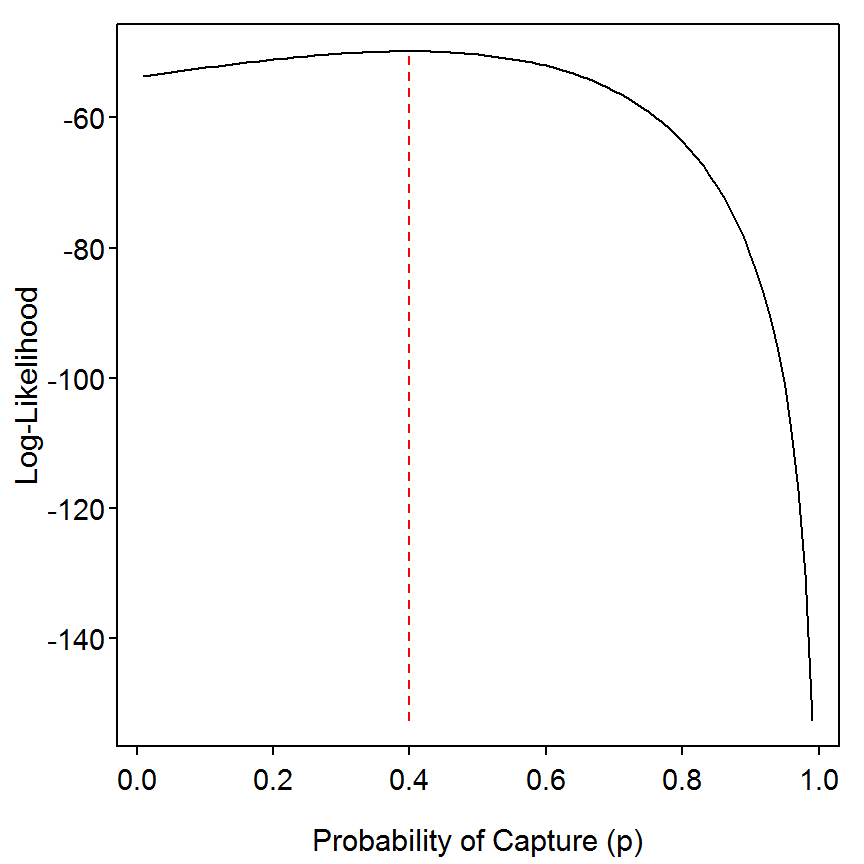> plot(plhs~ps,type="l",xlab="Probablity of Capture (p)",ylab="Log-Likelihood",ylim=c(-55,-49)) > lines(rep(phat,2),c(min(plhs),maxplh),lty=2,col="red")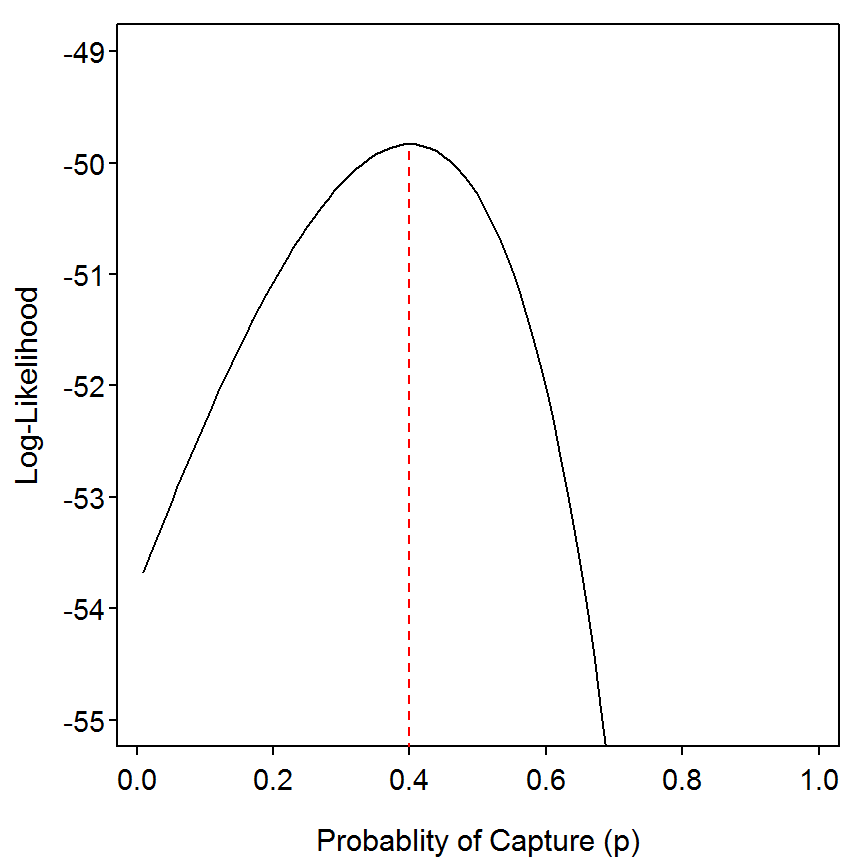### 8.5.4 Confidence Interval for the Probability of Capture The authors of the box note that a 95% confidence interval can be determined from the log-likelihood profile by finding the values of $$p$$ that correspond to the endpoints of the log-likelihood values that are 3.841 less than the maximum log-likelihood value. This process is generalized with the function defined below. > logLikCI <- function(logLiks,vals,conf.level=0.95) { rel.lhs <- max(logLiks)-logLiks CIrng <- vals[which(rel.lhs<qchisq(conf.level,1))] range(CIrng) } For example, the 95% CI for $$p$$ in this example is computed below. > ( phat.CI <- logLikCI(plhs,ps) )  0.01 0.65 ### 8.5.5 Maximizing the Log-Likelihood of $$N$$ The authors of the box show how $$N$$ is computed from the total catch in all removals (i.e., $$p$$) and what is essentially the denominator calculation in the likelihood parts given a value of $$p$$. Thus, this formula is used to compute $$N$$ for each value of $$p$$ in the sequence created above. The likelihood for each of the $$p$$s is then the likelihood for each of the corresponding $$N$$s and the optimal value for $$N$$ simply comes from the optimal value of $$p$$. The optimal value of $$N$$, likelihood profile (and plot), and likelihood profile confidence intervals are computed below. > ( Nhat <- n/(phat+phat*(1-phat)+phat*((1-phat)^2)) )  62.5 > Ns <- n/(ps+ps*(1-ps)+ps*((1-ps)^2)) > ( Nhat.CI <- logLikCI(plhs,Ns) )  51.19498 1649.77610 > plot(plhs~Ns,type="l",xlab="Population Size",ylab="Log-Likelihood") > lines(rep(Nhat,2),c(min(plhs),maxplh),lty=2,col="red")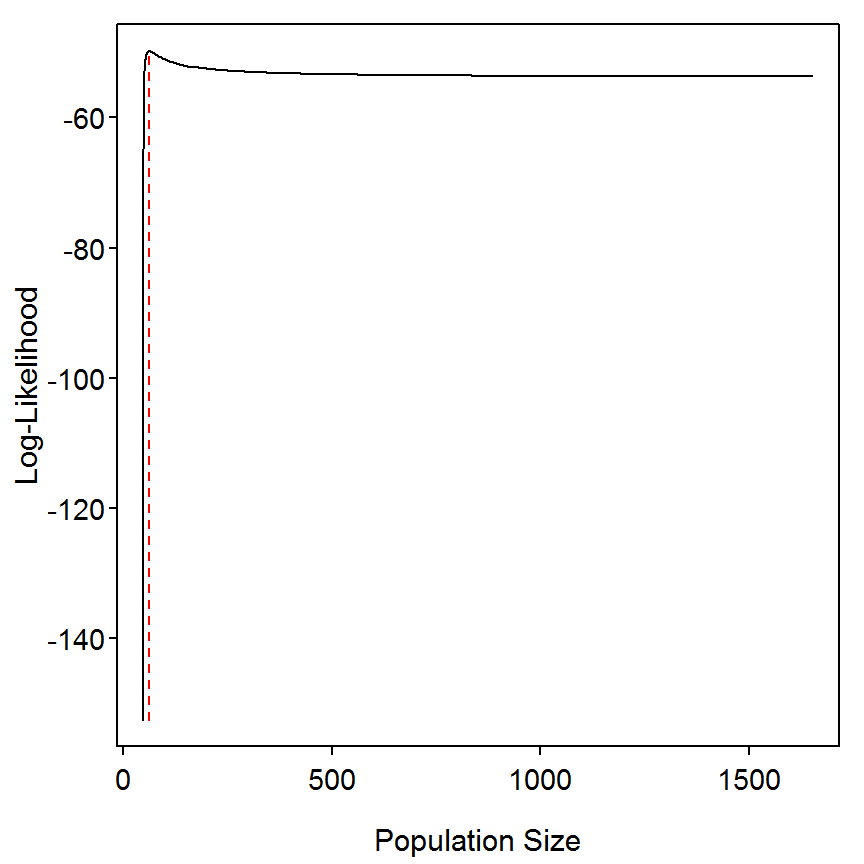> plot(plhs~Ns,type="l",xlab="Population Size",ylab="Log-Likelihood", ylim=c(-55,-49),xlim=c(50,400)) > lines(rep(Nhat,2),c(min(plhs),maxplh),lty=2,col="red")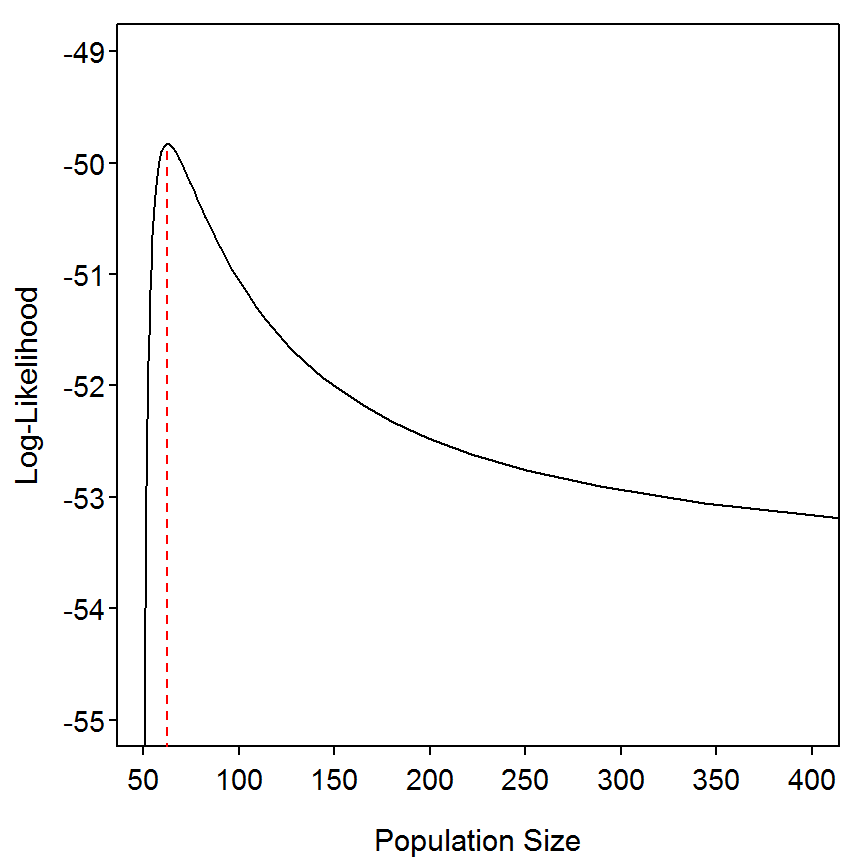### 8.5.6 Alternative Optimization Methods R provides several other methods for finding the minimum or maximum of a particular function (see the Optimization Vignette for an introduction). The optimize() function is most appropriate in this situation as the likelihood function to be maximized contains only one parameter. In this instance, optimize() requires the function to be maximized or minimized as the first argument, an interval over which to evaluate the parameter, the maximum=TRUE argument to force R to maximize rather than minimize (the default), and then remaining arguments to the function that is being minimized or maximized. Note that the first argument to the function to be minimized or maximized is the parameter that will be evaluated. > optim1 <- optimize(crmvlLH,interval=c(0,1),maximum=TRUE,catch=catch) > optim1$maximum      # p corresponding to maximum likelihood value
 0.4000003
> optim1$objective # maximum likelihood value  -49.83152 As an illustration, we could also use optim() but because $$p$$ must be between 0 and 1 we would use the "L-FBGS-B" optimization algorithm (again, see the Optimization portion of the Preliminaries Vignette for more information). The optimal value of $$p$$ is obtained with optim() below. > optim2 <- optim(0.2,crmvlLH,catch=catch,method="L-BFGS-B",lower=0.01,upper=0.99, control=list(fnscale=-1)) > optim2$par          # p corresponding to maximum likelihood value
 0.3999993
> optim2$value # maximum likelihood value  -49.83152 ### 8.5.7 Simplifying Function for 3-Pass Removal The removal() function is from the FSA package. The current version of removal() in the FSA package can only be used for 2- and 3-pass removal experiments. Experiments with more removals must use the more general technique described previously. is equipped to provide estimates of $$q$$ and $$N$$ for various methods applied to 3-pass and 2-pass removal data. This function requires a vector of catch data as the first argument and a method= argument that indicates which method will be used. The method described in the book (equations 8.22 and 8.23) for a three pass removal experiment is computed with method="Seber3" in removal(). The parameter estimates an resultant confidence intervals are extracted from the saved removal object with summary() and confint(), respectively. > rem <- removal(catch,method="Seber3") > summary(rem)  Estimate Std. Error No 62.5 10.486028 p 0.4 0.111851 > confint(rem) # normal approximation CI  95% LCI 95% UCI No 41.9477629 83.0522371 p 0.1807761 0.6192239 ## 8.6 Estimation of Abundance Based on the Removal Method in an Open Population A population of Lake Trout (Salvelinus namaycush) subjected to a commercial fishery was studied from 1985 to 2001 with the goal of determining trends in abundance over time. The population is sampled each year by a fishery-independent otter trawl survey. Data collected in the survey provide measures of relative abundance ($$\frac{C}{f}$$) for fish large enough to be vulnerable to capture in the commercial fishery (adults) and prerecruits that are not vulnerable to the commercial fishery. The number of fish harvested in the commercial fishery is recorded each year and is assumed to be occur at the beginning of the year. ### 8.6.1 Ogle Comment The authors of the box use Excel’s solver routine to find the optimal solution to a “hand-made” sum-of-squared-deviations formula. R provides a suite of optimization algorithms, but the algorithm used by Excel’s solver routine is not one of those in the R suite. The analysis demonstrated on this page will, thus, not exactly match that shown in the printed Box. However, there is no reason to believe that the solution provided by Excel’s solver is “better” in any regard than that provided by R’s optimization algorithms (or vice versa). You should read the Optimization portion of the Preliminaries Vignette for an introduction on using R’s optimization functions. ### 8.6.2 Preparing Data The Box8_6.txt data file is read and the structure is observed. > d6 <- read.table("data/Box8_6.txt",header=TRUE) > str(d6) 'data.frame': 17 obs. of 4 variables:$ year  : int  1985 1986 1987 1988 1989 1990 1991 1992 1993 1994 ...
$catch : int 94500 99154 74201 65827 66569 69000 93633 78069 78614 82258 ...$ rIndex: num  11.24 7.99 14.17 19.15 10.37 ...
$aIndex: num 43.1 38.5 29.7 32.9 35.1 ... ### 8.6.3 Optimization I The authors of the box set a constant fish mortality of 0.2. This should be saved to an object to allow ease of changing at a later time (e.g., if one were examining the sensitivity of the results to this assumption). The authors chose a starting value of 0.0001 for the catchability parameter and 1 for each of the “variability” parameters. In the code below I set each of these parameters separately and then combined them into one vector as R’s optimization routines expects all parameters to be in one vector. Note that rep() is used to create a vector with the first argument repeated the number of times shown in the second vector. Thus, starting values for the 35 parameters of this model are set as shown below. > M <- 0.2 # constant M > q <- 0.0001 # initial value for q > etas <- rep(1,length(d6$catch))       # use 1s as initial values for etas
> deltas <- rep(1,length(d6$catch)) # use 1s as initial values for deltas > par <- c(q,etas,deltas) # put all parameters into one vector The authors use a “sum-of-squared-errors” formula shown in the printed box on page 355. This formula requires some “prior” calculations shown by equations 8.34, 8.35, and 8.36 in the main text. These formulas are put into a function, called ABsse(), in the code below. This function requires the vector of parameter values as the first argument, the catch data as the second argument, the vector of adult cpe as the third argument, the vector of pre-recruit cpe as the four argument, and the assumed value of fishing mortality as the fifth argument. The function will return the SSE calculation shown in the box on page 355. > ABsse <- function(par,catch,A,R,M,sse.only=TRUE) { n <- length(catch) # get number of years of data q <- par # isolate q parameter eta <- par[2:(n+1)] # isolate eta parameters (begin 2nd, n long) delta <- par[(n+2):(2*n+1)] # isolate delta params (begin after eta, goto end) nhat <- A*eta # "True" adult index rhat <- R*delta # "True" recruitment index ntilde <- c(NA,(nhat[-n]-q*catch[-n]+rhat[-n])*exp(-M)) # compute N-tilde sse.1 <- sum(log10(eta)^2) # Sum of log etas sse.2 <- sum(log10(delta)^2) # Sum of log deltas sse.3 <- sum((A-ntilde)^2,na.rm=TRUE) # Sum of squared deviations Adult CPE sse <- sse.1+sse.2+sse.3 # compute overall SSE if (sse.only) sse else list(sse=sse,parts=c(sse.1,sse.2,sse.3),q, vals=data.frame(nhat=nhat,ntilde=ntilde,rhat=rhat,eta,delta)) } For example, the SSE calculation given the starting values is computed as shown in the first line below. The optimal values for the 35 parameters will be estimated with optim() as follows, > ABsse(par,catch=d6$catch,A=d6$aIndex,R=d6$rIndex,M=M)
 332.3245
> ABoptim1 <- optim(par,ABsse,catch=d6$catch,A=d6$aIndex,R=d6$rIndex,M=M) > ABoptim1$value                        # "minimum" SSE
 28.49486
> ABoptim1$counts # number of iterations function 502  > ABoptim1$convergence                  # a "1" means that maximum iterations limit was met
 1

However, the “convergence” message above indicates that the number of iterations was exceeded (which is fairly common with the default Nelder-Mead algorithm). The maximum number of iterations can be increased with the maxit= argument in the control= argument list.

> ABoptim2 <- optim(par,ABsse,catch=d6$catch,A=d6$aIndex,R=d6$rIndex,M=M, control=list(maxit=25000)) > ABoptim2$value                        # "minimum" SSE
 0.1583166
> ABoptim2$counts # number of iterations function 24640  > ABoptim2$convergence                  # a "0" means completed successfully
 0

Notice that several thousand more iterations were needed to arrive at an optimal solution but that solution had a dramatically lower SSE.

Let’s see if rescaling the parameters (see the Optimization portion of the Preliminaries Vignette) has a substantive impact on the optimization procedure. Notice that, with the rescaling of the parameters, that the number of iterations required to reach a solution was approximately 20% lower and that the SSE at the optimal solution is smaller. Thus, this appears to be a “better” solution than what was found above.

> par.scale <- c(1e-4,rep(1,length(d6$catch)),rep(1,length(d6$catch)))  # Set parameter scales
> ABoptim3 <- optim(par,ABsse,catch=d6$catch,A=d6$aIndex,R=d6$rIndex,M=M, control=list(parscale=par.scale,maxit=25000)) > ABoptim3$value                        # "minimum" SSE
 0.1364219
> ABoptim3$counts # number of iterations function 19758  > ABoptim3$convergence                  # a "0" means completed successfully
 0

### 8.6.4 Optimization II

The Nelder-Mead algorithm used above appears to require a large number of iterations to reach a “solution” and, just because it is the default method in R, it does not mean that it is the best method. The code below uses two other algorithms and shows the optimized SSE value for each.

> ABoptim4 <- optim(par,ABsse,method="BFGS",catch=d6$catch,A=d6$aIndex,R=d6$rIndex,M=M, control=list(parscale=par.scale,maxit=25000)) > ABoptim4$value
 0.08490653
> ABoptim4$counts function gradient 111 28  > ABoptim4$convergence
 0
> ABoptim5 <- optim(par,ABsse,method="CG",catch=d6$catch,A=d6$aIndex,R=d6$rIndex,M=M, control=list(parscale=par.scale,maxit=25000)) > ABoptim5$value
 0.08490653
> ABoptim5$counts function gradient 619 251  > ABoptim5$convergence
 0

Both functions compute a “so-called” gradient that helps the algorithm search for the minimum value. Thus, the “counts” output above shows the number of iterations to the main function first followed by the number of iterations to the gradient function. Note that both of the algorithms settled on a solution with a dramatically lower SSE than what the Nelder-Mead method found. The optimized SSEs for both algorithms were identical but the “BFGS” method used substantially fewer iterations. Thus, the “BFGS” provides the “best” result and would be the preferred results.

The optimal parameters are extracted (very carefully as you must remember what positions they are in the parameter vector).

> ( qhat <- ABoptim4$par )  0.000115052 > ( etahat <- ABoptim4$par[2:(length(d6$catch)+1)] )   1.0754067 1.0307863 1.1338227 0.9640450 1.1113685 0.9687304 1.1146669  0.8143174 1.2251303 0.7173742 0.8802057 1.0899432 1.3157643 1.0167209  0.9712818 1.1845740 1.0000005 > ( deltahat <- ABoptim4$par[(length(d6$catch)+2):(2*length(d6$catch)+1)] )
  1.0180895 1.0061669 1.0574785 0.9785676 1.0293410 0.9836605 1.0276695
 0.9021062 1.1932381 0.8735096 0.9068458 1.0477346 1.0273723 1.0122754
 0.9948431 1.0415007 1.0000005

The estimates of the number of adults (Nhat) and number of pre-recruits (Rhat) is estimated by re-arranging equations 8.34 and 8.35, respectively, and using the parameter estimates computed above.

> ( Nhat <- d6$aIndex*etahat/qhat )   403328.9 344575.0 292689.7 275257.1 338765.9 289477.3 338221.1 219766.3  252688.7 231388.9 175349.5 222532.1 317584.8 252563.0 321560.0 329883.4  298213.1 > ( Rhat <- d6$rIndex*deltahat/qhat )
   99462.20  69875.13 130240.85 162879.12  92777.75 150132.78  85034.71
 110242.44 219871.42  94220.47 134388.98 120480.54  31253.72 185822.54
  57069.53  79208.79  78312.45

Note, as a general rule-of-thumb I would use all three main algorithms in optim() to find “optimal” solutions and then choose the results with the most “optimal” solution.

### 8.6.5 Comparison to Results in Box 8.6

The results shown in the box (actually taken from the Excel spreadsheet provided on the book CD) can be loaded from the Box8_6res.txt data file.

> res <- read.table("data/Box8_6res.txt",header=TRUE)
> str(res)
'data.frame':   17 obs. of  5 variables:
$year : int 1985 1986 1987 1988 1989 1990 1991 1992 1993 1994 ...$ Nhat    : int  404106 345247 293215 275807 339441 290035 338783 220131 2530..
$Rhat : int 99766 70078 130682 163343 93111 150535 85313 110506 220645 9..$ etahat  : num  1.075 1.03 1.133 0.963 1.111 ...
$deltahat: num 1.019 1.006 1.058 0.979 1.03 ... The parameter values can be sent to ABsse() to see how the SSE from the “best-fit” model from Excel’s solver routine compares to the BFGS results above. It can be seen that the BFGS results found a solution with a slightly smaller SSE. > parbox <- c(0.0001147,res$etahat,res$deltahat) > ABsse(parbox,catch=d6$catch,A=d6$aIndex,R=d6$rIndex,M=M)
 0.08636522

The effect of these different parameter findings on estimates of adult and pre-recruit abundance can be seen by combining the BFGS results from above with the results from the Box into one table. From this it appears that the results shown here provide estimates that are approximately -0.2% lower for adults and -0.3% lower for pre-recruits. Effectively the results from using the BFGS optimization algorithm here match the results from the Excel solver routine used to produce the box.

> compTbl <- data.frame(year=d6$year,NhatBFGS=round(Nhat,0),NhatBook=res$Nhat,
Npdiff=(round(Nhat,0)-res$Nhat)/res$Nhat*100,RhatBFGS=round(Rhat,0),
RhatBook=res$Rhat, Rpdiff=(round(Rhat,0)-res$Rhat)/res$Rhat*100) > round(compTbl,1)  year NhatBFGS NhatBook Npdiff RhatBFGS RhatBook Rpdiff 1 1985 403329 404106 -0.2 99462 99766 -0.3 2 1986 344575 345247 -0.2 69875 70078 -0.3 3 1987 292690 293215 -0.2 130241 130682 -0.3 4 1988 275257 275807 -0.2 162879 163343 -0.3 5 1989 338766 339441 -0.2 92778 93111 -0.4 6 1990 289477 290035 -0.2 150133 150535 -0.3 7 1991 338221 338783 -0.2 85035 85313 -0.3 8 1992 219766 220131 -0.2 110242 110506 -0.2 9 1993 252689 253001 -0.1 219871 220645 -0.4 10 1994 231389 231912 -0.2 94220 94364 -0.2 11 1995 175350 175706 -0.2 134389 134730 -0.3 12 1996 222532 223029 -0.2 120481 120793 -0.3 13 1997 317585 318281 -0.2 31254 31410 -0.5 14 1998 252563 253196 -0.3 185823 186325 -0.3 15 1999 321560 322324 -0.2 57070 57199 -0.2 16 2000 329883 330591 -0.2 79209 79522 -0.4 17 2001 298213 299049 -0.3 78312 78541 -0.3 ## 8.7 Application of Surplus Production Modeling The commercial fishery for a population of Alewife (Alosa pseudoharengus) was monitored from 1985 to 2001. Each year, the total weight of the catch (catch) and the total effort (effort) were recorded, providing $$\frac{C}{f}$$ as a measure of relative abundance. These data were analyzed using a surplus production model to estimate carrying capacity ($$K$$), initial biomass ($$B_{0}$$), catchability ($$q$$), and the intrinsic rate of growth ($$r$$) for this fishery population. ### 8.7.1 Preparing Data The Box8_7.txt data file is read, the structure is observed, and a new variable containing the catch-per-unit-effort is appended to the data frame. > d7 <- read.table("data/Box8_7.txt",header=TRUE) > str(d7) 'data.frame': 17 obs. of 3 variables:$ year  : int  1985 1986 1987 1988 1989 1990 1991 1992 1993 1994 ...
$effort: int 825 1008 1411 1828 2351 2074 1877 1566 1139 893 ...$ catch : int  90000 113300 155860 181128 198584 198395 139040 109969 71896 5..
> d7$cpe <- d7$catch/d7$effort ### 8.7.2 Optimization I The authors identified starting value for the initial biomass (B0), carrying capacity (K), catchability (q), and intrinsic rate of increase (r). In the code below I set each of these parameters separately and then combined them into one vector as R’s optimization routines expects all parameters to be in one vector. In this case I also named the parameters in the vector as the function I derive below requires named parameters (this allows some flexibility in the ordering of the parameters). > B0 <- 800000 # initial value of B0 > K <- 1000000 # initial value of K > q <- 0.0001 # initial value of q > r <- 0.17 # initial value of r > pars <- c(B0,K,q,r) # put all parameters into one vector > names(pars) <- c("B0","K","q","r") # name the parameters The authors use a “sum-of-squared-errors” formula to find the optimal parameters for this surplus production model. This formula requires some “prior” calculations shown in the Box. These formulas are put into a function, called SPsse(), in the code below. This function requires the vector of parameter values as the first argument, the “biomass” data as the second argument, and the CPE data as the third argument. The function will return the SSE value given the parameters and data. > SPsse <- function(par,B,CPE,SSE.only=TRUE) { ## This repeats Excel calculations n <- length(B) # get number of years of data B0 <- par["B0"] # isolate B0 parameter K <- par["K"] # isolate K parameter q <- par["q"] # isolate q parameter r <- par["r"] # isolate r parameter predB <- numeric(n) predB <- B0 for (i in 2:n) predB[i] <- predB[i-1]+r*predB[i-1]*(1-predB[i-1]/K)-B[i-1] predCPE <- q*predB sse <- sum((CPE-predCPE)^2) if (SSE.only) sse else list(sse=sse,predB=predB,predCPE=predCPE) } For example, the SSE at the starting parameter values is computed below. > ( res1 <- SPsse(pars,d7$catch,d7$cpe) )  551805.4 As the starting values for the parameters are several orders of magnitude different I immediately created a vector to rescale the parameters and then used the default Nelder-Mead algorithm to find optimize the SSE function. > pars.scale <- c(1e5,1e6,1e-4,1e-1) # set rescale values for parameters > SPoptim1 <- optim(pars,SPsse,control=list(maxit=10000,parscale=pars.scale),B=d7$catch,CPE=d7$cpe) > SPoptim1$value                                # "minimum" SSE
 1618.429
> SPoptim1$counts # number of iterations function 929  > SPoptim1$convergence                          # a "0" means completed successfully
 0

### 8.7.3 Optimization II

I then tried both the “BFGS” and “CG” algorithms to see if they found a “better” solution (i.e., lower SSE). As can be seen below, the “CG” algorithm did not converge to a solution.

> SPoptim2 <- optim(pars,SPsse,method="BFGS",control=list(maxit=10000,parscale=pars.scale),
B=d7$catch,CPE=d7$cpe)
> SPoptim2$value # "minimum" SSE  1618.428 > SPoptim2$counts                               # number of iterations
function gradient
587      139 
> SPoptim2$convergence # a "0" means completed successfully  0 > SPoptim3 <- optim(pars,SPsse,method="CG",control=list(maxit=10000,parscale=pars.scale), B=d7$catch,CPE=d7$cpe) In this instance the Nelder-Mead and “BFGS” algorithms resulted in essentially the same minimum SSE value without any major differences in number of iterations. Thus, I will use the results from the default Nelder-Mead algorithm. The optimal parameters are extracted from that model with, > SPoptim1$par                                  # the optimal parameter estimates
          B0            K            q            r
7.322595e+05 1.158451e+06 1.485687e-04 4.057310e-01 

### 8.7.4 Plot of Observed and Predicted CPE Over Time

The SSE.only=FALSE argument to SPsse() will cause the function to also return the predicted biomass and predicted cpe values. These values are found for the optimal solution with the code below. A plot comparing the predicted and observed cpe values over time is then constructed.

> res3 <- SPsse(SPoptim1$par,d7$catch,d7$cpe,SSE.only=FALSE) > str(res3) List of 3$ sse    : num 1618
$predB : num [1:17] 732260 751562 745365 697343 628833 ...$ predCPE: num [1:17] 108.8 111.7 110.7 103.6 93.4 ...
> plot(cpe~year,data=d7,pch=19,xlab="Year",ylab="CPE")
> lines(d7$year,res3$predCPE,lwd=2,col="red")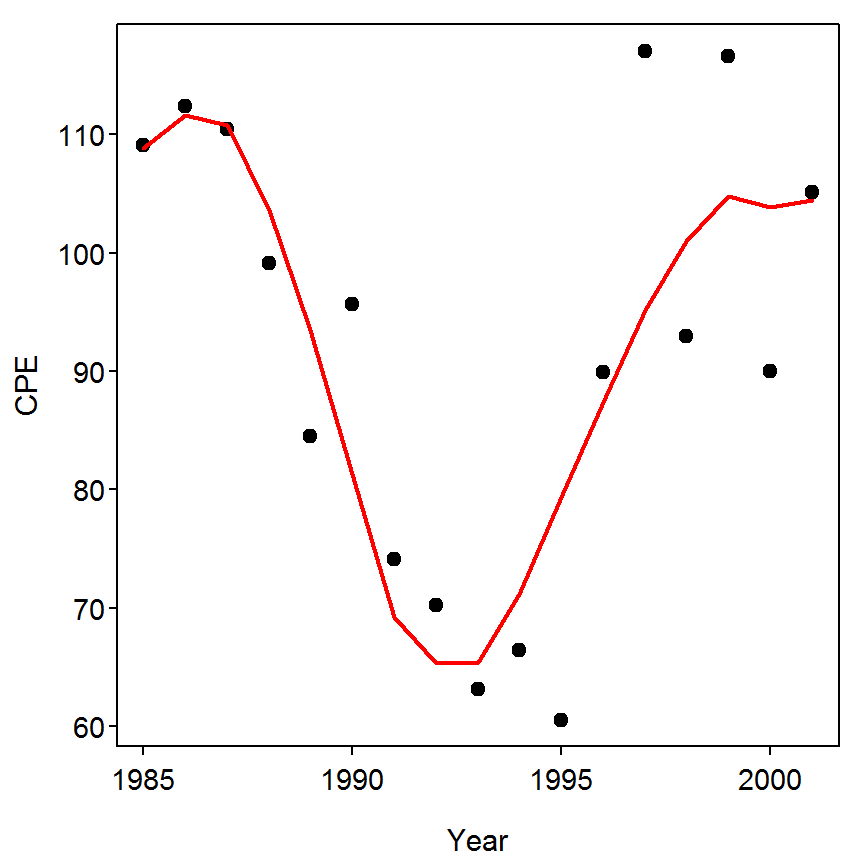### 8.7.5 Comparison to Results in Box 8.7

The optimal parameters found with Excel solver and shown in the box are entered into a vector and then sent to SPsse() to compute the SSE given the data and those parameter values. As can be seen below, the SSE given the parameter values here and in the box are essentially the same. The parameter estimates are, however, slightly different.

> parsbox <- c(732506,1160771,0.0001484,0.4049) # put all parameters into one vector
> names(parsbox) <- c("B0","K","q","r")         # name the parameters
> res2 <- SPsse(parsbox,d7$catch,d7$cpe,SSE.only=FALSE)
> res2$sse  1618.438 > cbind(SPoptim1$par,parsbox)
                     parsbox
B0 7.322595e+05 7.325060e+05
K  1.158451e+06 1.160771e+06
q  1.485687e-04 1.484000e-04
r  4.057310e-01 4.049000e-01

The estimates of B and CPE and the percent differences are computed below. Thus, effectively, the results from using the Nelder-Mead optimization algorithm here match the results from the Excel solver routine used in the Box.

> compTbl <- data.frame(year=d7$year,BNM=round(res3$predB,0),BBox=round(res2$predB,0), CPENM=round(res3$predCPE,1),CPEBox=round(res2$predCPE,1)) > compTbl  year BNM BBox CPENM CPEBox 1 1985 732260 732506 108.8 108.7 2 1986 751562 751933 111.7 111.6 3 1987 745365 745867 110.7 110.7 4 1988 697343 697954 103.6 103.6 5 1989 628833 629503 93.4 93.4 6 1990 546892 547577 81.3 81.3 7 1991 465636 466305 69.2 69.2 8 1992 439582 440225 65.3 65.3 9 1993 440288 440902 65.4 65.4 10 1994 479136 479719 71.2 71.2 11 1995 533818 534369 79.3 79.3 12 1996 588301 588829 87.4 87.4 13 1997 640434 640961 95.1 95.1 14 1998 679637 680190 101.0 100.9 15 1999 705005 705608 104.7 104.7 16 2000 698953 699622 103.8 103.8 17 2001 703187 703911 104.5 104.5 > pdiffTbl <- data.frame(year=d7$year,Bpdiff=(compTbl$BNM-compTbl$BBox)/compTbl$BBox*100, CPEpdiff=(compTbl$CPENM-compTbl$CPEBox)/compTbl$CPEBox*100)
> round(pdiffTbl,1)
   year Bpdiff CPEpdiff
1  1985    0.0      0.1
2  1986    0.0      0.1
3  1987   -0.1      0.0
4  1988   -0.1      0.0
5  1989   -0.1      0.0
6  1990   -0.1      0.0
7  1991   -0.1      0.0
8  1992   -0.1      0.0
9  1993   -0.1      0.0
10 1994   -0.1      0.0
11 1995   -0.1      0.0
12 1996   -0.1      0.0
13 1997   -0.1      0.0
14 1998   -0.1      0.1
15 1999   -0.1      0.0
16 2000   -0.1      0.0
17 2001   -0.1      0.0

## 8.8 Production Estimation Based on the Instantaneous Growth Rate Method

Density, mean weight, and biomass (and associated variances) of a Brook Trout (Salvelinus fontinalis) population in Valley Creek, Minnesota, were estimated in a stream reach with an area of 0.181 ha on four dates between March 1974 and March 1975 (Waters 1999). Population statistics for two of these dates are presented below in order to illustrate how to estimate production using the instantaneous growth rate method.

### 8.8.1 Ogle Comment

The computations of Box 8.8 form an example of the increment summation method for computing production. Special purpose software (called Pop/Pro and made available with a description from here) has been developed to automate these calculations for more realistically complex situations. This document will follow the example in the box using R, but it should be noted that this is basically using R as a calculator. More complex examples should use the Pop/Pro software.

### 8.8.2 Preparing Data

The Box8_8.txt data file is read and the structure is observed. The natural log of the mean weight variable (i.e., meanw) will ultimately be needed in the calculations below and is added to the data frame. The variance of the log mean weight is given in the main text with equation 8.51 and is also added to the data frame

> d8 <- read.table("data/Box8_8.txt",header=TRUE)
> str(d8)
'data.frame':   8 obs. of  8 variables:
$date : Factor w/ 2 levels "29Jul74","8Mar74": 2 2 2 2 1 1 1 1$ age      : int  1 2 3 4 1 2 3 4
$dens : num 277.9 157.5 36.1 11.2 276.4 ...$ var.dens : num  1336 317.7 34 13.2 553.6 ...
$meanw : num 6.86 28.56 107.23 170.05 24.27 ...$ var.meanw: num  0.13 0.79 19.89 42.09 0.62 ...
$B : num 1905 4500 3872 1899 6709 ...$ var.B    : num  75456 222126 469765 350364 306075 ...
> d8$logmeanw <- log(d8$meanw)
> d8$var.logmeanw <- d8$var.meanw/(d8$meanw^2) The two sample times were then separated into individual data frames using Subset(), which requires the original data frame as the first argument and a conditioning statement as the second argument. > ( d8a <- Subset(d8,date=="8Mar74") )  date age dens var.dens meanw var.meanw B var.B logmeanw var.logmeanw 1 8Mar74 1 277.85 1336.05 6.86 0.13 1905.34 75455.97 1.925707 0.0027624544 2 8Mar74 2 157.54 317.71 28.56 0.79 4499.83 222126.45 3.352007 0.0009685247 3 8Mar74 3 36.11 34.00 107.23 19.89 3872.13 469764.75 4.674976 0.0017298250 4 8Mar74 4 11.17 13.16 170.05 42.09 1898.89 350364.34 5.136093 0.0014555451 > ( d8b <- Subset(d8,date=="29Jul74") )  date age dens var.dens meanw var.meanw B var.B logmeanw var.logmeanw 1 29Jul74 1 276.45 553.56 24.27 0.62 6709.17 306074.53 3.189241 1.052573e-03 2 29Jul74 2 68.08 94.58 77.31 2.49 5262.90 278582.66 4.347823 4.166084e-04 3 29Jul74 3 9.76 7.64 146.18 100.67 1427.00 167558.97 4.984839 4.711120e-03 4 29Jul74 4 8.12 1.11 194.72 1.67 1582.12 30259.81 5.271563 4.404487e-05 ### 8.8.3 Calculations “By Hand” The equations from page 363 in the main text and Box 8.8 are then coded as below. > meanB <- (d8a$B+d8b$B)/2 > var.meanB <- (d8a$var.B+d8b$var.B)/4 # equation 8.49 > G <- d8b$logmeanw-d8a$logmeanw # given below equation 8.47 > var.G <- d8a$var.logmeanw + d8b$var.logmeanw # equation 8.50 > P1 <- meanB*G # equation 8.47 > var.P1 <- var.meanB*(G^2)+var.G*(meanB^2) # equation 8.48 > cbind(d8a$age,meanB,var.meanB,G,var.G,P1,var.P1) # make a table for display purposes only
          meanB var.meanB         G       var.G        P1     var.P1
[1,] 1 4307.255  95382.62 1.2635336 0.003815027 5442.3613 223058.077
[2,] 2 4881.365 125177.28 0.9958162 0.001385133 4860.9422 157136.597
[3,] 3 2649.565 159330.93 0.3098627 0.006440945  821.0013  60514.827
[4,] 4 1740.505  95156.04 0.1354701 0.001499590  235.7864   6289.112

Note that the units of P1 in this case are grams per sampled reach. It was noted in the box that the sampled reach represented 0.181 ha. Thus, if the P1 values from above are divided by 0.181 then the spatial units will be hectares and if divided by 1000 the weight units will be kg for a final set of units of kg/ha. Notice that if the mean is divided by 0.181 and 1000 then the corresponding variance must be divided by the square of these constants (as noted in the box).

> ( P <- P1/0.181/1000 )
 30.068295 26.856034  4.535919  1.302687
> var.P <- var.P1/(0.181^2)/(1000^2)

As noted in the box, approximate confidence intervals for $$P$$ are constructed using standard normal theory.

> conf.level <- 0.95
> ( z <- qnorm(1-(1-conf.level)/2) )
 1.959964
> me <- z*sqrt(var.P)
> P.lci <- P-me
> p.uci <- P+me
> round(cbind(d1$age,P,var.P,me,P.lci,p.uci),3)  P var.P me P.lci p.uci [1,] 30.068 6.809 5.114 24.954 35.183 [2,] 26.856 4.796 4.292 22.564 31.149 [3,] 4.536 1.847 2.664 1.872 7.200 [4,] 1.303 0.192 0.859 0.444 2.161 The overall production estimate with margin-of-error and confidence intervals is then computed as below. > Ptotal <- sum(P) > var.Ptotal <- sum(var.P) > me.Ptotal <- z*sqrt(var.Ptotal) > Ptotal.lci <- Ptotal-me.Ptotal > Ptotal.uci <- Ptotal+me.Ptotal > round(cbind(Ptotal,var.Ptotal,me.Ptotal,Ptotal.lci,Ptotal.uci),3)  Ptotal var.Ptotal me.Ptotal Ptotal.lci Ptotal.uci [1,] 62.763 13.644 7.24 55.523 70.003 ### 8.8.4 Allen Curves Allen curves plot the density of individuals versus mean individual weight. This plot is constructed for the two time frames with, > plot(dens~meanw,data=d8a,type="b",pch=19,col="red",xlab="Mean Individual Weight", ylab="Density",xlim=c(0,max(d8a$meanw,d8b$meanw)),ylim=c(0,max(d8a$dens,d8b$dens))) > points(dens~meanw,data=d8b,type="b",pch=19,col="blue") > legend("topright",legend=c("8Mar74","29Jul74"),pch=19,col=c("red","blue"),lwd=1)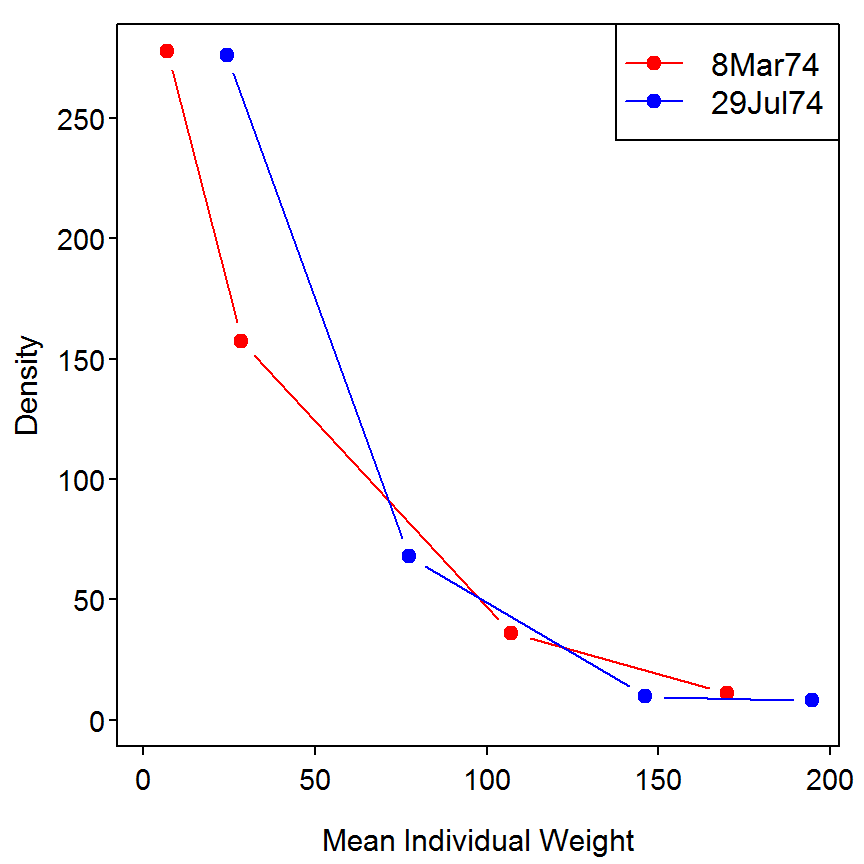## 8.9 Production Estimation Based on the Size-Frequency Method Density and mean weight (and associated variances) of a Rainbow Trout (Oncorhynchus mykiss) population in Valley Creek, Minnesota, were estimated in a stream reach on three dates between April 1977 and April 1978 (Garman and Waters 1983). The catch data were broken into 10 size-groups in order to allow investigators to estimate production using the size-frequency method. ### 8.9.1 Preparing Data The Box8_9.txt data file is read and the structure is observed. > d9 <- read.table("data/Box8_9.txt",header=TRUE) > str(d9) 'data.frame': 10 obs. of 5 variables:$ lenGroup: int  1 2 3 4 5 6 7 8 9 10
$meanN : num 260.2 281.7 144.9 88.8 67.7 ...$ meanw   : num  3.2 6.9 12.6 27.9 49.9 ...
$varN : num 2653.5 1491.4 182.5 145.9 49.5 ...$ varw    : num  0.2 0.1 0.1 1.4 5.1 45 24.8 11.8 39.9 13.1

### 8.9.2 Estimation of P “By Hand”

First, set the number of length groupings and the CPI value (given in box) to objects for later use.

> c <- length(d9$meanN) > CPI <- 3  Equation 8.52 is code below. The first three lines below are used to find the “difference” in mean densities in the middle summation portion in the brackets of 8.52. The line corresponding to Ndiff1 and Ndiff2 are the differences in mean density portions of the first and last term in the brackets of 8.52. These values are then put together into a single vector for later use. > temp1 <- d9$meanN[1:(c-2)]
> temp2 <- d9$meanN[3:c] > Ndiff2 <- temp1-temp2 > Ndiff1 <- d9$meanN-d9$meanN > Ndiff3 <- d9$meanN[c-1]-d9$meanN[c] > ( Ndiff <- c(Ndiff1,Ndiff2,Ndiff3) )   -21.5 115.3 192.9 77.2 45.7 11.8 16.2 36.1 11.9 4.8 The final result of equation 8.52, i.e., the estimate of production ($$P$$), is then computed. The difference between this result and the result shown in the box is completely due to the fact that I divide by 3 here whereas the author of the box multiplied by 0.333. > ( Phat1 <- 0.5*c*sum(d9$meanw*Ndiff)/CPI )
 32614.13

The variance of $$P$$ is computed below using a similar “trick” as above. Again, note that the difference in the result here and in the box is due to dividing by three or multiplying by 0.333.

>  # note the "plus" in the first term below
> wdiff <- c(d9$meanw+d9$meanw,d9$meanw[1:(c-2)]-d9$meanw[3:c],d9$meanw[c-1]-d9$meanw[c])
> ( var.Phat1 <- ((0.5*c)^2)*sum((wdiff^2)*d9$varN+d9$varw*(Ndiff^2))/(CPI^2) )
 18351993

The estimated $$P$$ and variance are converted to kilograms (rather than grams) per hectare per year by dividing the estimate by 1000 and the variance by 1000 squared.

> Phat <- Phat1/1000
> var.Phat <- var.Phat1/(1000^2)

An approximate confidence interval for $$P$$ is computed with standard normal theory.

> conf.level <- 0.95
> ( z <- qnorm(1-(1-conf.level)/2) )
 1.959964
> me <- z*sqrt(var.Phat)
> P.lci <- Phat-me
> p.uci <- Phat+me
> round(cbind(Phat,var.Phat,me,P.lci,p.uci),3)
       Phat var.Phat    me  P.lci p.uci
[1,] 32.614   18.352 8.396 24.218 41.01

Reproducibility Information
Compiled Date: Wed May 13 2015
Compiled Time: 9:31:15 PM
Code Execution Time: 20.41 s

R Version: R version 3.2.0 (2015-04-16)
System: Windows, i386-w64-mingw32/i386 (32-bit)
Base Packages: base, datasets, graphics, grDevices, grid, methods, stats,
utils
Required Packages: FSA, Rcapture and their dependencies (car, dplyr,
gdata, gplots, graphics, Hmisc, knitr, lmtest, multcomp, plotrix,
relax, sciplot, stats)
Other Packages: car_2.0-25, estimability_1.1, Formula_1.2-1, FSA_0.6.13,
lattice_0.20-31, lsmeans_2.17, multcomp_1.4-0, mvtnorm_1.0-2,
NCStats_0.4.3, nlstools_1.0-1, plotrix_3.5-11, Rcapture_1.4-2,
rmarkdown_0.6.1, survival_2.38-1, TH.data_1.0-6
boot_1.3-16, caTools_1.17.1, cluster_2.0.1, coda_0.17-1,
codetools_0.2-11, colorspace_1.2-6, DBI_0.3.1, digest_0.6.8,
dplyr_0.4.1, evaluate_0.7, foreign_0.8-63, formatR_1.2, gdata_2.16.1,
gplots_2.17.0, gridExtra_0.9.1, gtable_0.1.2, gtools_3.4.2, highr_0.5,
htmltools_0.2.6, KernSmooth_2.23-14, latticeExtra_0.6-26, lme4_1.1-7,
lmtest_0.9-33, magrittr_1.5, MASS_7.3-40, Matrix_1.2-0, mgcv_1.8-6,
minqa_1.2.4, munsell_0.4.2, nlme_3.1-120, nloptr_1.0.4, nnet_7.3-9,
parallel_3.2.0, pbkrtest_0.4-2, plyr_1.8.2, proto_0.3-10,
quantreg_5.11, RColorBrewer_1.1-2, Rcpp_0.11.6, relax_1.3.15,
reshape2_1.4.1, rpart_4.1-9, sandwich_2.3-3, scales_0.2.4,
sciplot_1.1-0, SparseM_1.6, splines_3.2.0, stringi_0.4-1,
stringr_1.0.0, tools_3.2.0, yaml_2.1.13, zoo_1.7-12

## References

Garman, G. C., and T. F. Waters. 1983. Use of the size-frequency (Hynes) method to estimate annual production of a stream fish population. Canadian Journal of Fisheries and Aquatic Sciences 40:2030–2034.

Otis, D. L., K. P. Burnham, G. C. White, and D. R. Anderson. 1978. Statistcal inference from capture data on closed animal populations. Wildlife Monographs 62:1–135.

Waters, T. F. 1999. Long-term trout production dynamics in Valley Creek, Minnesota. Transactions of the American Fisheries So 128:1151–1162.